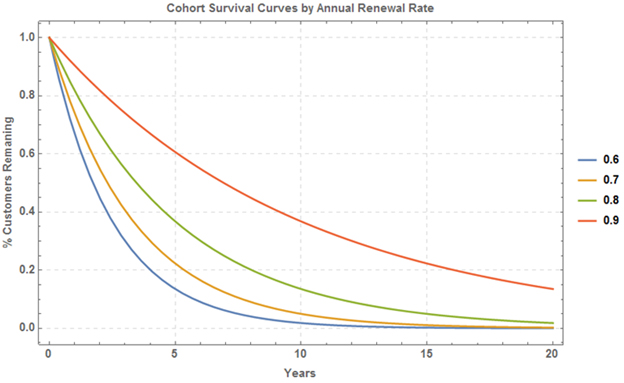## Average Customer Length

Mark Coleman, Jeffrey Wu

“How long does a typical customer stay with us?” is a straightforward question, but answering it proves to be a rather intricate problem. This question is in the same realm of attempting to figure out the Lifetime Value of a customer or LTV. There is no direct, easy way of measuring this, but there a ways to help us estimate it. We will cover a couple approaches to estimate the answer.

### Renewal Rate Estimate

As you may know, the quick rule of thumb is that the average or expected lifetime of a customer can be estimated as the reciprocal of the churn rate. For instance, if 20% of our customers churn out each year, then the expected lifetime of a customer is 1/0.20 = 5 years. So where does this come from?

Imagine we have a cohort of customers who have a continuous and constant retention rate of r % each time period, say a year. Under this assumption, the size of the size of the active cohort will decay following a smooth curve, e.g., something like this:The chart above shows how the size of a cohort declines over time under different renewal assumptions. The green line corresponds to an annual renewal rate of 80% – the behavior of our earlier example. As it turns out, the assumption of this sort of constant decay can be modeled using the so-called exponential distribution. The exponential distribution is extensively used in certain branches of mathematics, such as queueing theory and survival analysis. It is also used in physics. Remember the idea of a half-life? The same math applies here.

The exponential distribution is defined with respect to one parameter, let’s call it p, that defines how quickly the curve declines (you may recall that the more familiar normal distribution is defined with two parameters, the mean and variance). The parameter p is in fact our churn rate, p = 1 - r. The expected value of the exponential distribution, its mean, is simply 1/p. Thus with a retention rate of 80%, churn is 20%, and the corresponding expected lifetime is 1/20% = 5 years. If retention is 75%, then annual churn is 25%, and the corresponding customer lifetime is 1/25% = 4 years. Higher churn implies lower lifetimes. To see this more clearly, we can let retention vary and then draw customer lifetime as a function of retention:A Technical Note: Using the exponential distribution, we are implicitly assuming that customers churn out at a continuous rate. If we assume that customers churn out discretely, say only at the end of each year, then we get a slightly different set of results. Using an analogy from finance, its equivalent to calculating interest payments using continuous compounding versus discrete compounding.

### Survival Distribution Estimate

This approach is a bit more rigorous than using the renewal rate method rule of thumb. In this method, we are modeling the time to which a specific event occurs, in this case a customer churning. However, this method also allows us account for the customers who are still active customers (more technically known as right censored data). The result of this method yields what’s called a survival curve, which we can graph. This is shown below by plotting the percentage of customers who are still active after a period of time:This chart represents the entire customer base of our fictitious company. For example, after being a customer for one year, ~82% of all customers still continue to be a customer, regardless of when they actually started. Another way to interpret this chart is that out of all our customers ever, 32% of them remain active customers today.

Building this allows us to calculate various statistics about the survival rate of the overall population. We estimate the customer lifetime calculating the expected value from the distribution, which is more commonly called the median (median = 5 years in our example).